# Integration¶

This chapter doesn’t assume any knowledge about differential geometry. The most versatile way to do integration over manifolds is explained in the differential geometry section.

## General Case¶

We want to integrate a function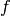over a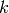-manifold in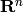, parametrized as: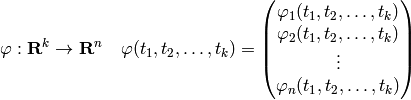then the integral of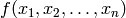over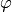is: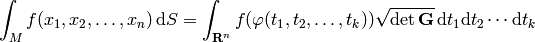where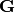is called a Gram matrix and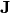is a Jacobian: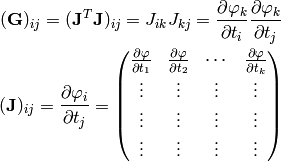The idea behind this comes from the fact that the volume of the-dimensional parallelepiped spanned by the vectors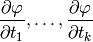is given by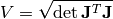whereis an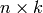matrix having those vectors as its column vectors.

### Example¶

Let’s integrate a function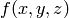over the surface of a sphere in 3D (e.g.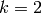and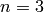):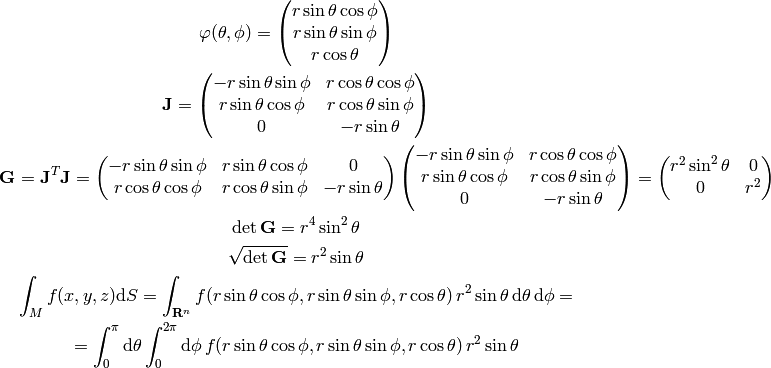Let’s say we want to calculate the surface area of a sphere, so we set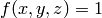and get: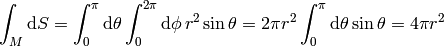## Special Cases¶

### k = n¶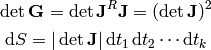### k = 1¶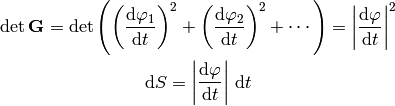### k = n - 1¶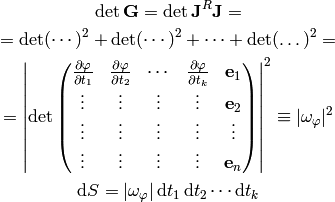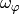is a generalization of a vector cross product. The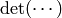symbol means a determinant of a matrix with one row removed (first term in the sum has first row removed, second term has second row removed, etc.).

### k = 2, n = 3¶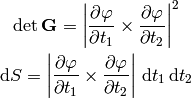### y = f(x, z)¶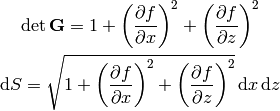in general for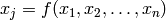we get: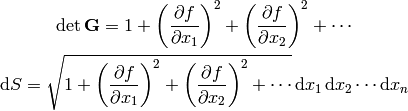The “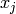” term is missing in the sums above.

### Implicit Surface¶

For a surface given explicitly by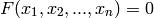we get: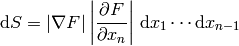### Orthogonal Coordinates¶

If the coordinate vectors are orthogonal to each other: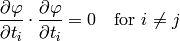we get: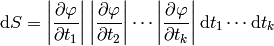## Motivation¶

Let the-dimensional parallelepiped P be spanned by the vectors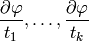and letismatrix having these vectors as its column vectors. Then the area of P isso the definition of the integral over a manifold is just approximating the surface by infinitesimal parallelepipeds and integrating over them.

## Example¶

Let’s calculate the total distance traveled by a body in 1D, whose position is given by: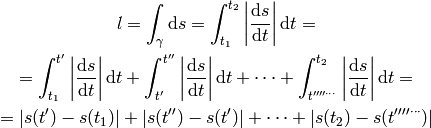where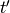,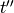, ... are all the points at which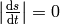, so each of the integrals in the above sum has either positive or negative integrand.